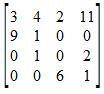High School Trigonometry: Homeschool Curriculum Final Exam

Free Practice Test Instructions:

Choose your answer to the question and click 'Continue' to see how you did. Then click 'Next Question' to answer the next question. When you have completed the free practice test, click 'View Results' to see your results. Good luck!

Question 4 4. The following graph is of a polynomial function of degree 4. Which of the following best describes the solutions?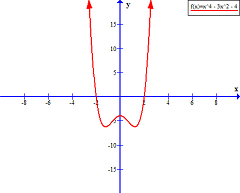Question 5 5. Evaluate f(f(x)). [Yes, it says f(f(x)]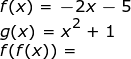Question 6 6. If arc AC is 122 degrees and arc BC is 192 degrees, what is the measure of angle ACB?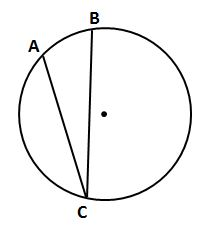Question 8 8. In order to complete the square on the following equation, what value would you add to both sides?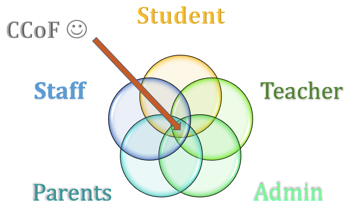Question 9 9. What is the line, or axis, of symmetry of the following parabola?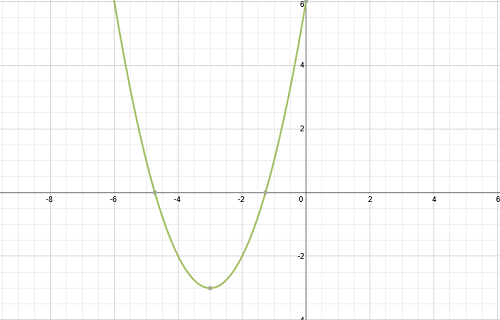Question 11 11. Find angle A.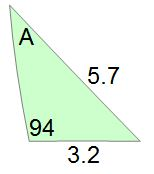Question 13 13. Which of the following is the new row that results when you add rows 1 and 3?